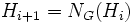# HN-group

This article defines a group property: a property that can be evaluated to true/false for any given group, invariant under isomorphism
View a complete list of group properties
VIEW RELATED: Group property implications | Group property non-implications |Group metaproperty satisfactions | Group metaproperty dissatisfactions | Group property satisfactions | Group property dissatisfactions

This article defines a term that has been used or referenced in a journal article or standard publication, but may not be generally accepted by the mathematical community as a standard term.[SHOW MORE]
The version of this for finite groups is at: finite HN-group

## History

### Origin

The term HN-group or hypernormalizing group was introduced by Alan R. Camina as part of his Ph.D. thesis in 1967. His first paper on the subject appeared in Math, Z. 100, Pages 59 - 68.

## Definition

### Symbol-free definition

A group is termed an HN-group or hypernormalizing group if the hypernormalizer of any ascendant subgroup is the whole group, or equivalently if every ascendant subgroup is hypernormalized.

For a finite group, this is equivalent to demanding that the hypernormalizer of any subnormal subgroup be the whole group, or equivalently, that any subnormal subgroup is finitarily hypernormalized.

### Definition with symbols

A group$G$ is termed an HN-group or hypernormalizing group if for any ascendant subgroup$H$, the hypernormalizer of$H$, viz the limit of the normalizer sequence for$H$ in$G$, is the whole of$G$.

A finite group$G$ is termed an HN-group if for any subnormal subgroup$H$, the sequence$H_i$ where$H_0 = H$ and$H_{i+1} = N_G(H_i)$ reaches$G$ in finitely many steps.

## Formalisms

### In terms of the subgroup property collapse operator

This group property can be defined in terms of the collapse of two subgroup properties. In other words, a group satisfies this group property if and only if every subgroup of it satisfying the first property (ascendant subgroup) satisfies the second property (hypernormalized subgroup), and vice versa.
View other group properties obtained in this way

We can define a HN-group as a group for which ascendant subgroup = hypernormalized subgroup

For finite groups, this is the same as saying subnormal subgroup = hypernormalized subgroup